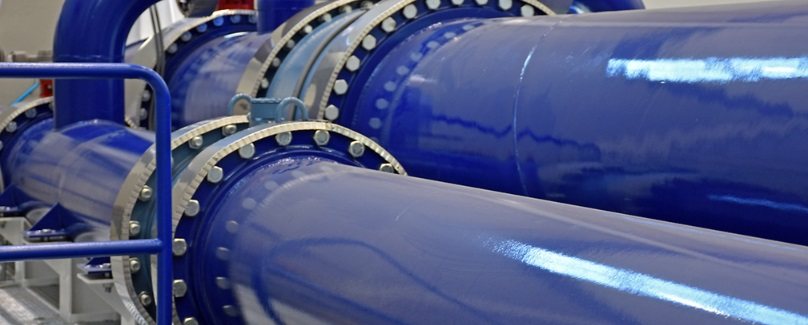# Overview of flow measurement.## Introduction

The ability to measure flow rate is another important process measurement. There are four different classes of flow measurement transducers: differential pressure measurement, velocity-based measurement, positive displacement measurement, and mass flow measurement.

Differential pressure measurement devices can be separated into restrictive and non-restrictive devices. When fluid flows through a restrictive flow element, there is a differential pressure (P1-P2) across that element. This pressure is directly related to volume flow rate. Since we are measuring a differential pressure, most any of the above pressure
transducers may be used to detect that pressure. There are four types of sensors based on this principle: orifice plates, venturi tubes, flow nozzles and wedge elements. The advantages and disadvantages of each are shown in the table below.Nonrestrictive devices include pitot-static tubes and annubars. In each case, the pressure transmitted is a function of the fluid velocity. Of course, if one knows fluid velocity, one may apply the continuity equation to determine the volume flow rate. The problem with the pitot-static tube is it only measures velocity at one point, usually the center line. However, since the velocity of flow is variable along the cross-section with a maximum occurring at the center line, the resulting signal only indicates center line velocity, not average velocity. In theory, we could develop a relationship between this maximum and center line velocity, but this tends to be difficult to do in practice. To deal with this problem, we use an annubar. Operating on the same principle as the pitot-static tube, the annubar detects the average velocity pressure across the cross-section of the duct or pipe.

### Turbine Type Flow Meter

Velocity based flow detection is accomplished with a turbine or paddlewheel sensor, a vortex shedding sensor, a magnetic sensor, or an ultrasonic sensor. The turbine/paddlewheel sensor places a rotating element into the flow
stream. As the element rotates, it rotates by a magnetic pickup resulting in a very low voltage pulse. A transmitter converts this pulse into a standard process signal### Vortex Shedding Flow Meter

When fluid flows over an object, the flow separates from the object at certain points. If the object is properly shaped, the way the flow separates is predictable. As the flow separates, a vortex is formed. This vortex exerts a pressure different from the local static pressure and proportional to fluid flow velocity.### Magnetic flowmeter

A magnetic flowmeter works on the basis of fundamental electrical and magnetic laws. In such a meter, the fluid acts as the conductor. The meter itself is constructed of a nonconductive material. Surrounding the meter is a series of electrical coils and sensor pickups. The electrical coils are used to generate a magnetic field. As the fluid moves through the magnetic field, a voltage is induced in the fluid. The sensors detect the magnitude of this voltage, which
is proportional to the velocity of the fluid flow

### Doppler Type Flow Meter

The ultrasonic meter may be either Doppler effect or time-of-travel meters. The time-of-travel meter uses a series of
sound producing elements to generate a sonic signal. They are arranged in such a fashion that the velocity of the fluid is proportional to the velocity of the transmitted sound as well as the frequency of that sound. The Doppler
meter relies on suspended particles or bubbles in the fluid to reflect sound waves generated by the device. It works
on the same principle as police radar.### positive displacement meter

The positive displacement meter is a rotary device based on positive displacement pumps. Although there are a
number of configurations, they all work in essentially the same manner. All types of these meters entrap a specified volume of fluid. The fluid is forced through the meter by the differential pressure across the meter. The meter is designed such that fluid flow through the meter causes a shaft to rotate or oscillate. Volume flow is then proportional to the rotational speed of this shaft. Figures illustrate two of the more common PD flow meters.### coriolis mass flow meter

When highly precise measurement is required, the use of the coriolis mass flow meter is warranted. One configuration of the mass flow meter is a U-shaped tube as shown in Figure . The tube is placed into vibration around the y-axis by an electromagnet. As fluid passes through the vibrating tube, a force imbalance causes the
tube to twist around the x-axis. A pair of electromagnetic detectors detects the twist. The degree of twist is proportional to the mass flow rate through the device. These meters can handle a very wide range of flows and are extremely accurate and repeatable. However, they are quite costly and generally require significant space for installation. They are also unable to handle aerated flows and two-phase flows.